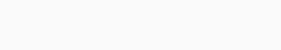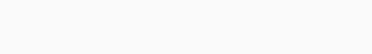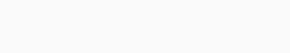# CA Foundation Business Economics Study Material – Production Optimisation - Learn Hool

#### CA Foundation Business Economics Study Material Chapter 3 Theory of Production and Cost – Production Optimisation

Production Optimisation
Isoquants:

An iso-product curve or isoquant is a curve, which represents the various combinations of two variable inputs that give the same level of output. As all combinations on the iso-product curve give the same level of output, the producer becomes indifferent to these combinations. That is why iso-product curve are also called ‘production indifference curve’ or ‘equal product curve’. To understand consider the following production isoquant schedule.In the schedule I above, the producer is indifferent whether he gets combination A, B, C, D or E. This is because all the combinations of capital and labour give the same level of output i.e. 100 units.

By plotting the above combinations on a graph, we can derive an iso-product curve as shown in the following figure:In the diagram, quantity of capital is measured on X-axis and quantity of labour on Y-axis.

The various combinations A, B, C, D, E of capital and labour are plotted and on joining them we derive an iso-product curve. All combinations lying on the iso-product curve yield the same level of output i.e. 100 units and hence technically equally efficient.

If the production schedule II is also plotted on the graph, we will get another iso-product curve IQ200. This will lie above the IQ100 as the combinations contain greater quantities of capital and labour. A set of iso-product curves is called iso-product curve map.In the diagram, it can be observed that each iso-product curve is labelled in terms of output. All combinations lying of IQ100 give the output of 100 units and all the combinations lying on IQ200 give the output of 200 units. Higher iso-product curve represent higher level of output. Also it indicates how much more output can be achieved.

Marginal Rate of Technical Substitution
The rate at which one factor of production is substituted in place of the other factor without any change in the level of output is called as the marginal rate of technical substitution. Consider the following schedule.Each of the factor combinations in the table above yields same level of output. Moving from combination A to B, one unit of capital replaces 4 units of labour. Similarly, moving from B to C, one unit of capital now replaces only 3 units of labour and so on. It implies that labour and capital are imperfect substitutes. That is why MRTSKL is continuously diminishing. We can measure MRTSKL on an iso-product curve.

‘Iso-Cost Line’ OR ‘Equal Cost Lines’
Iso-cost line (also known Equal Cost Line; Price Line; Outlay Line; Factor Price Line) shows the various combinations of two factor inputs which the firm can purchase with a given outlay (i.e. budget) and at given prices of two inputs.

Example. A firm has with itself Rs. 1,000 which it would like to spend on factor ‘X’ and factor ‘Y’.
Price of factor ‘X’ is Rs. 20 per unit.
Price of factor ‘Y’ is Rs. 10 per unit.
Therefore, if the firm spends the whole amount on factor X, it can buy 50 units of X and if the whole amount is spent on factor Y, it can buy 100 units of Y. However, in between these two extreme limits, it can have many combinations of X and Y for the outlay of Rs. 1,000. Graphically it can be shown as follows –In the diagram OP shows 100 units of Y and OM shows 50 units of X. When we join the two points P and M, we get the iso-cost line. All the combinations of factor X and factor Y lying on iso-cost line can be purchased by the firm with an outlay of Rs. 1,000. If the firm increases the outlay to Rs. 2,000, the iso-cost line shifts to the right, if prices of two factors remains unchanged. The slope of the iso-cost line is equal to the ratio of the prices of two factors. Thus,Producer’s Equilibrium OR Production Optimization
A firm always try to produce a given level of output at minimum cost. For this it has to use that combination of inputs which minimizes the cost of production. This ensures maximization of profits and produce a given level of output with least cost combination of inputs. The least-cost combination of inputs or factors is called producer’s equilibrium or production optimization. This is determined with the help of (a) isoquants, & (b) iso-cost line.

An isoquant or iso-product curve is a curve which shows the various combinations of two inputs that produce same level of output. The isoquants are negatively sloped and convex to origin. The slope of isoquants shows the marginal rate of technical substitution which diminishes. Thus, MRTSxyIso-cost line shows the various combination of two factor inputs which the firm can purchase with a given outlay and at given prices of inputs. There can be different outlays and hence different iso-cost lines. Slope of iso-cost line shows the ratio of the price of two inputs i.e. Px/PyWhich will be the least cost combination can be understood with the help of following figure. Suppose firm wants to produce 300 units of a commodity. It will first see the isoquant that represents 300 units.

In the adjoining diagram we find that all combinations a, b, c, d and e can produce 300 units of output. In order to produce 300 units firm with try to find out least cost combination. For this it will super impose the various iso-cost lines on isoquant as shown in the diagram. The diagram shows that combination ‘C’ is,the least cost combination as here isoquant is tangent to iso-cost line HI. All other combinations a, b, d and e lying on isoquant cost more as these points lie on higher iso-cost lines. Hence, the point of tangency of isoquant and iso-cost line shows least cost combination. At the point of tangency.

Slope of iso-quant = Slope of iso-cost lineThus, the firm will choose OM units of factor X and ON units of factor Y and be at equilibrium as the marginal physical products of two factors are proportional to the factor prices.

@ Designed By : Vikas Copyright 2023 @ LearnHool.In